# Trend Channels

Trend channels are used to track the momentum of a trend, with

• peaks (or highs) frequently respecting the upper trend channel; and
• troughs (or lows) respecting the lower trend channel.

They are useful for setting stop loss orders and for swing trading. There are several different trend channel options on the Draw toolbar: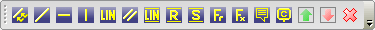These are also found on the Draw menu.

### Auto-Fit Trend Channel

Automatically fits a trendline above or below the selected data (depending on where you drag the channel line) and draws a parallel line through the opposite extreme point.

To draw an auto-fit trend channel:

• Select Trend Channel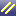on the Draw toolbar.
• Click and drag above (down-trend) or below (up-trend) the selected data.• Select Closing Price for long-term charts or Highs and Lows for short-term.
• The trend channel will be drawn automatically:### Linear Regression Trend Channel

Automatically fits a linear regression line to the selected data, and draws parallel lines through the extreme points on each side of the linear regression line.Follow the same sequence as for Auto-Fit Trend Channels, clickingon the toolbar. It makes no difference whether you drag your mouse above or below the selected data.

### Raff Regression Channel

Automatically fits a linear regression line to the selected data, draws a parallel line through the most extreme point from the linear regression line, then draws a second parallel line at equal distance on the opposite side of the linear regression line.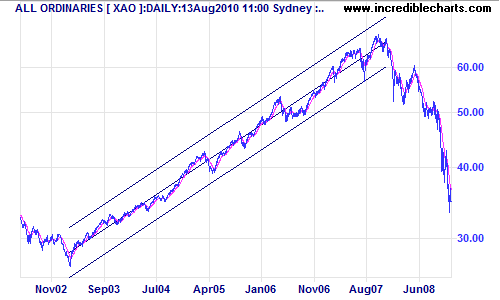Clickon the toolbar and set up the same as for Linear Regression Trend Channels.

### Standard Deviation Channel

Standard deviation channels are parallel lines drawn around a linear regression line at a set number of standard deviations.Follow the same procedure as for Linear Regression Channels, clicking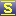on the toolbar and selecting the number of standard deviations (usually 2).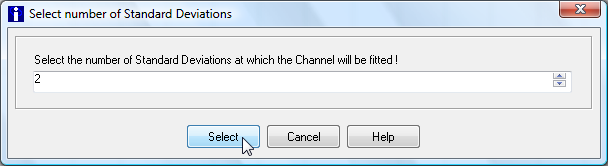## Editing A Trend Channel

Trend Channels are saved as individual lines, and can be modified or deleted independently (as separate, normal trendlines).

## Trend Channels: Evaluation

Standard Deviation Channels seem the most useful trend channel option. They can be set to exclude the extreme data points that often result in other trend channels being drawn too wide.

Using 2 standard deviations encloses roughly 95% of the selected data; 3 standard deviations encloses about 99% of the selected data but closely resembles the Raff Regression Channel.Two sets of standard deviation channels are drawn on the above chart:

• 2 standard deviations (dark)
• 3 standard deviations (light)

You can see that the dark trend channels (at 2 standard deviations) more accurately represent trend action for the period and offers better signals.